Next: Power of test statistics Up: Basic principles of time Previous: Test statistics

Corrections to the probability distribution

In principle, it is possible to compute a value of the statistic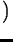for a single frequency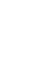and to test its consistency with a random signal (Ho). The common procedure of inspecting the whole periodogram for a detected signal corresponds to the N-fold repetition of the single test for a set of trial frequencies,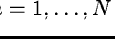. The probability of the whole periodogram being consistent with Ho is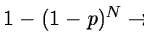for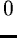. The factor N means that there is an increased probability of accepting a given value of the statistic as consistent with a random signal. Therefore, increasing the number of trial frequencies decreases the sensitivity for the detection of a significant signal and accordingly is called the penalty factor for multiple trials or for the frequency bandwidth used. The true number of independent frequencies, Nt, remains generally unknown. It is usually less than the number of resolved frequencies(Sect. 12.3.1) because of aliasing and still less than the number of computed frequencies Nc, because of oversampling: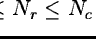. For a practical and conservative estimate, we recommend to use Nr as the number of trial frequencies, N.

According to the standard null hypothesis, Ho, the noise is white noise. This is not the case in many practical cases. For instance, often the noise is a stochastic process with a certain correlation length lcorr>0, so that on average ncorr consecutive observations are correlated. Such noise corresponds to white noise passed through a low pass filter which cuts off all frequencies above 1/lcorr. Such correlation is not usually taken into account by standard test statistics. The effect of this correlation is to reduce the effective number of observations by a factor ncorr(Schwarzenberg-Czerny, 1989). This has to be accounted for by scaling both the statistics S and the number of its degrees of freedom njby factors depending on ncorr.

In the test statistic, a continuum level which is inconsistent with the expected value of the statistic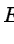may indicate the presence of such a correlation between consecutive data points. A practical recipe to measure the correlation is to compute the residual time series (e.g. with the SINEFIT/TSA command) and to look for its correlation length with COVAR/TSA command. The effect of the correlation in the parameter estimation is an underestimation of the uncertainties of the parameters; the true variances of the parameters are a factor ncorr larger than computed.

In the command individual descriptions, we often refer to probability distributions of specific statistics. For the properties of these individual distributions see e.g. Eadie et. al. (1971), Brandt (1970), and Abramovitz & Stegun (1972). The two latter references contain tables. For a computer code for the computation of the cumulative probabilities see Press et. al. (1986).Next: Power of test statistics Up: Basic principles of time Previous: Test statistics
Petra Nass
1999-06-15